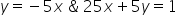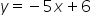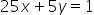Maths-
General
Easy

Question

# Are graphs of the equations parallel, perpendicular or neither?Hint:

## The correct answer is: slope are equal then both are parallel.

### We have been given two equations in the question for which we have to tell are graphs of the equations parallel, perpendicular or neither. Step 1 of 1:We have given two equationsSlope of both lines are -5, -5 respectivelySince slope are equal then both are parallel.#### With Turito Foundation.#### Get an Expert Advice From Turito.